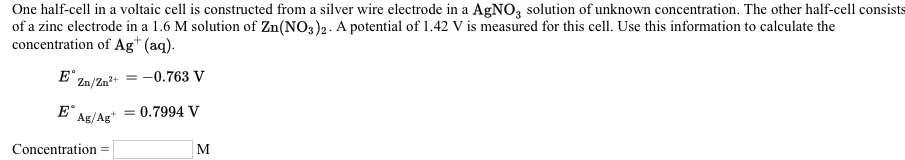# One half-cell in a voltaic cell is constructed from a silver wire electrode in a AgNO3 solution of unknown concentration. The other half-cell consists of a zinc electrode in a 1.6 M solution of Zn(NO3)2. A potential of 1.42 V is measured for this cell. Use this information to calculate the concentration of Ag^+(aq). E° Zn/Zn^2+ = -0.763 V E° Ag/Ag^+ = 0.7994 V